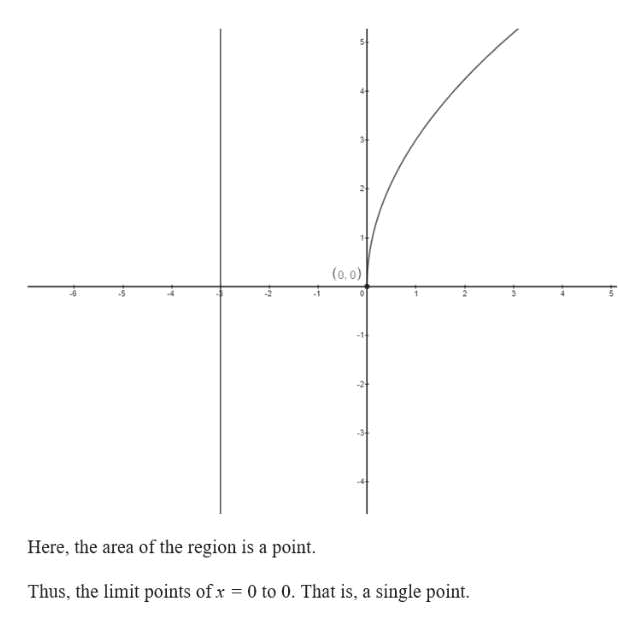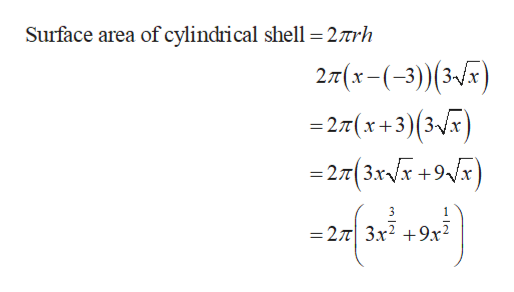# Use the method of cylindrical shells to find the volume V generated by rotating the region bounded by the given curves about the specified axis.y = 3sqrt(x); y = 0; x = 0     Rotating around x = -3;

Question
32 views

Use the method of cylindrical shells to find the volume V generated by rotating the region bounded by the given curves about the specified axis.

y = 3sqrt(x); y = 0; x = 0     Rotating around x = -3;
check_circle

Step 1

Find the volume of the solid obtained by rotating region as follows.

Step 2

Use online graphing calculator to draw the functions as follows.help_outlineImage Transcriptionclose(0,0) Here, the area of the region is a point. Thus, the limit points of x 0 to 0. That is, a single point fullscreen
Step 3

Find the area of the re...help_outlineImage TranscriptioncloseSurface area of cylindrical shell =27rh 2T(x-(-3)) (3-) =27(x+3) (3x) = 27t( 3XVX +9Vx 3 1 =27 3x2 9x2 fullscreen

### Want to see the full answer?

See Solution

#### Want to see this answer and more?

Solutions are written by subject experts who are available 24/7. Questions are typically answered within 1 hour.*

See Solution
*Response times may vary by subject and question.
Tagged in

### Integration